﻿ 基于QBVMD的变压器励磁涌流与故障识别研究 Research on Transformer Inrush Current and Fault Identification Based on QBVMD

Smart Grid
Vol. 10  No. 04 ( 2020 ), Article ID: 37131 , 6 pages
10.12677/SG.2020.104021

Research on Transformer Inrush Current and Fault Identification Based on QBVMD

Xiaodong Yan1, Qingfu Kong1, Jianming Sun1, Di Pan1, Weili Wu2, Jun Liu3

1Hami Power Supply Company of State Grid Xinjiang Electric Power Co., Ltd., Hami Xinjiang

2Xi’an University of Science and Technology, Xi’an Shaanxi

3Anhui Zhengguang Electric Power Technology Co., Ltd., Hefei AnhuiReceived: Jul. 31st, 2020; accepted: Aug. 13th, 2020; published: Aug. 20th, 2020ABSTRACT

In order to solve the problem that transformer differential protection is easy to be disturbed by inrush current, this paper proposes an identification method of inrush current based on variational mode decomposition and optimized neural network. Using PSCAD software platform to build transformer inrush current and fault current model, obtain inrush current data, usequasi-bi- VMD to decompose differential current signal, obtain different scale signal, then use optimized neural network to identify fault type. Finally, the transformer magnetizing inrush current, reactive inrush current, single-phase grounding and three-phase grounding fault scenarios are set up and the corresponding fault data are obtained. The results show that the proposed method can accurately identify transformer inrush current and fault current, and the recognition accuracy is high, which provides a reference for identifying transformer inrush current and fault.

Keywords:Inrush Current, Transformer, QBVMD, Optimized Neural Network1国网新疆电力有限公司哈密供电公司，新疆 哈密

2西安科技大学，陕西 西安

3安徽正广电电力技术有限公司，安徽 合肥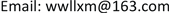1. 引言

2. 基于QBVMD的涌流数据特征提取

$\underset{\left\{{u}_{k}\right\},\left\{{\omega }_{k}\right\}}{\mathrm{min}}\left\{\underset{k}{\sum }‖{\partial }_{t}\left[\left(\delta \left(t\right)+\frac{j}{\pi t}\right)\ast {u}_{k}\left(t\right)\right]{\text{e}}^{-j{w}_{k}t}‖\right\}$ (1)

$\text{s}\text{.t}\text{.}\text{\hspace{0.17em}}\text{\hspace{0.17em}}\underset{k=1}{\overset{k}{\sum }}{u}_{k}=f\left(t\right)$ (2)

$\left[\begin{array}{cccc}x\left(1\right)& x\left(1+\tau \right)& \cdots & x\left(1+\left(m-1\right)\tau \right)\\ ⋮& ⋮& & ⋮\\ x\left(j\right)& x\left(j+\tau \right)& \cdots & x\left(j+\left(m-1\right)\tau \right)\\ ⋮& ⋮& & ⋮\\ x\left(G\right)& x\left(G+\tau \right)& \cdots & x\left(G+\left(m-1\right)\tau \right)\end{array}\right]$ (3)

$PE\left(m\right)=-\underset{i=1}{\overset{G}{\sum }}{P}_{t}\mathrm{ln}{P}_{t}$ (4)

$PE=PE\left(m\right)/\mathrm{ln}\left(m!\right)$ (5)

3. 算例分析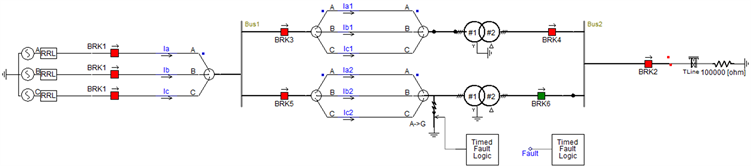Figure 1. Transformer inrush current and fault simulation model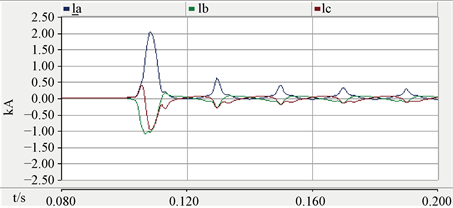(a)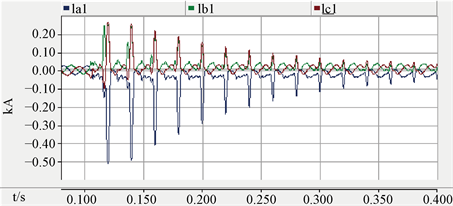(b)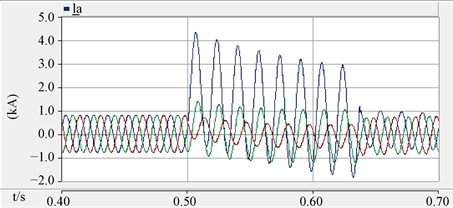(c)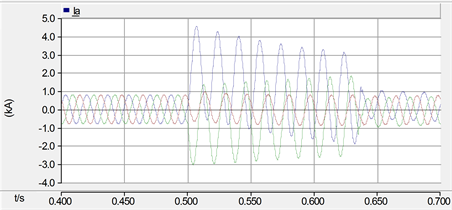(d)

Figure 2. Fault signal. (a) Inrush current waveform of transformer no-load closing; (b) Transformer and inrush waveform; (c) Single phase short circuit current waveform; (d) Phase to phase short circuit fault waveform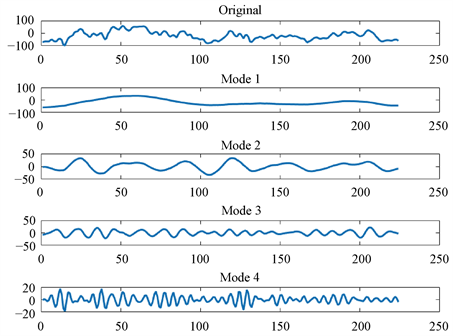Figure 3. VMD extraction process (1)Figure 4. VMD extraction results (2)Table 2. Transformer inrush current and fault identification after adding white noise

4. 结语

Research on Transformer Inrush Current and Fault Identification Based on QBVMD[J]. 智能电网, 2020, 10(04): 190-195. https://doi.org/10.12677/SG.2020.104021

1. 1. 李沐. 基于直流励磁及选相控制的变压器励磁涌流抑制技术研究[D]: [硕士学位论文]. 济南: 山东大学, 2020.

2. 2. 王伟, 魏菊芳, 方琼, 等. 变压器3种励磁涌流抑制措施效果比较[J]. 高压电器, 2020, 56(2): 101-107.

3. 3. 周博曦, 秦晋, 王金亮, 等. 基于人工神经网络与有限状态机的变电站告警处理系统[J]. 山东电力技术, 2020, 47(266): 6-13.

4. 4. 陈仕镇. 基于神经网络和有限状态机的游戏AI决策引擎设计与实现[D]: [硕士学位论文]. 厦门: 厦门大学, 2016.

5. 5. 鲁阳. 海上风电系统故障预测与视情维修方法研究[D]: [博士学位论文]. 哈尔滨: 哈尔滨工程大学, 2018.

6. 6. Wang, W., Pan, C. and Wang, J. (2018) Quasi-Bivariate Variational Mode Decomposition as a Tool of Scale Analysis in Wall-Bounded Turbulence. Experiments in Fluids, 59, 1.
https://doi.org/10.1007/s00348-017-2450-7

7. 7. 杨大为, 冯辅周, 赵永东, 等. VMD样本熵特征提取方法及其在行星变速箱故障诊断中的应用[J]. 振动与冲击, 2018, 37(16): 198-205.

8. 8. 郑小霞, 周国旺, 任浩翰, 等. 基于变分模态分解和排列熵的滚动轴承故障诊断[J]. 振动与冲击, 2017, 36(22): 22-28.

9. 9. 艾澍海, 张寿明. 基于VMD和形态差值滤波器的特征提取算法[J]. 传感器与微系统, 2018(9): 151-154.

10. 10. 曹风华. 基于改进禁忌算法和ANN的故障诊断推理[J]. 计算机系统应用, 2012(11): 165-169.